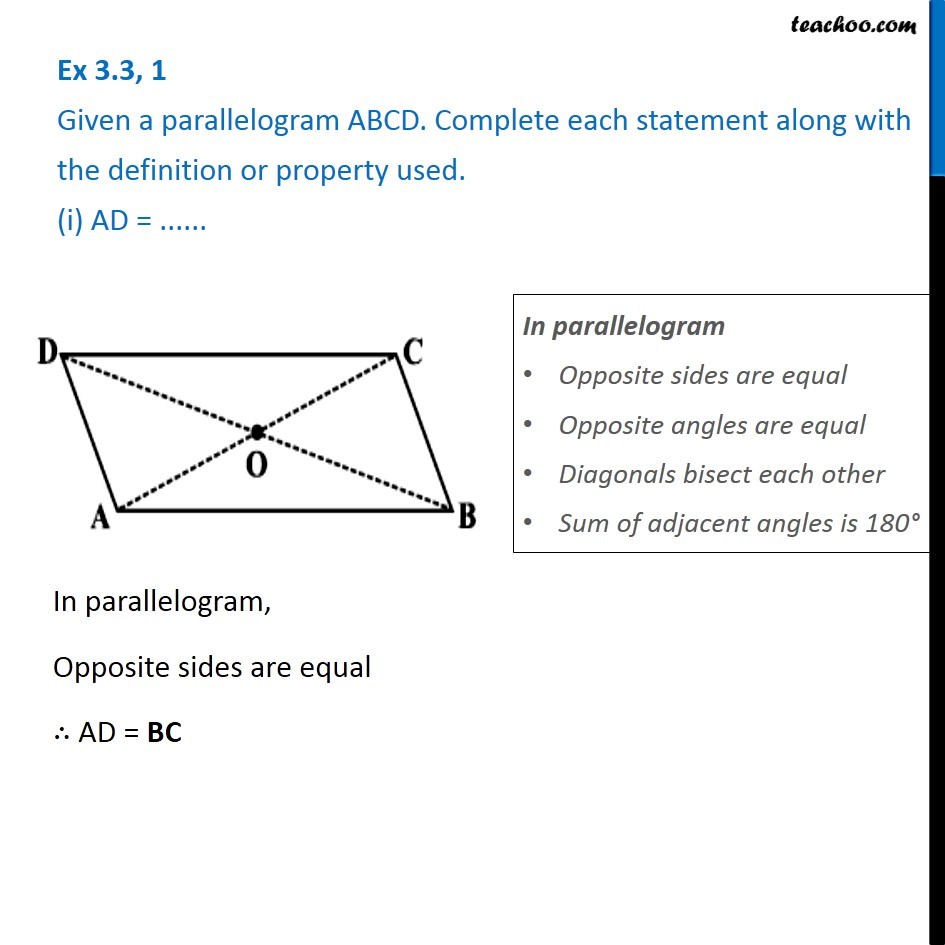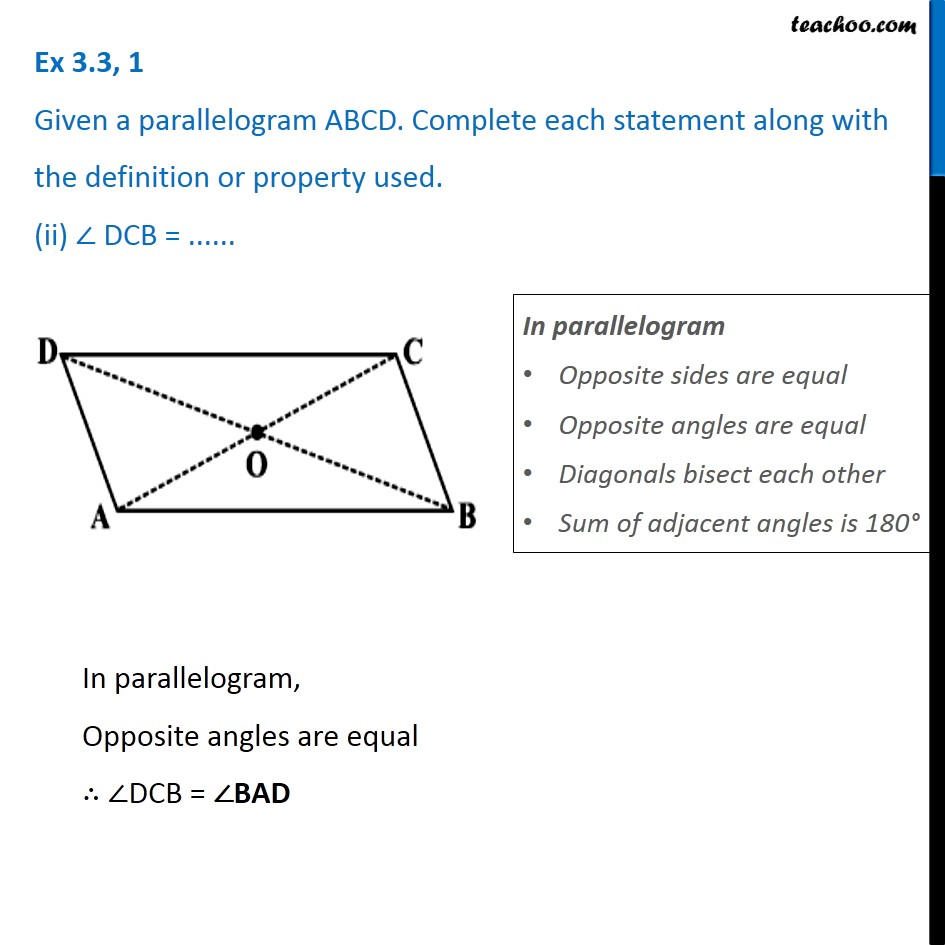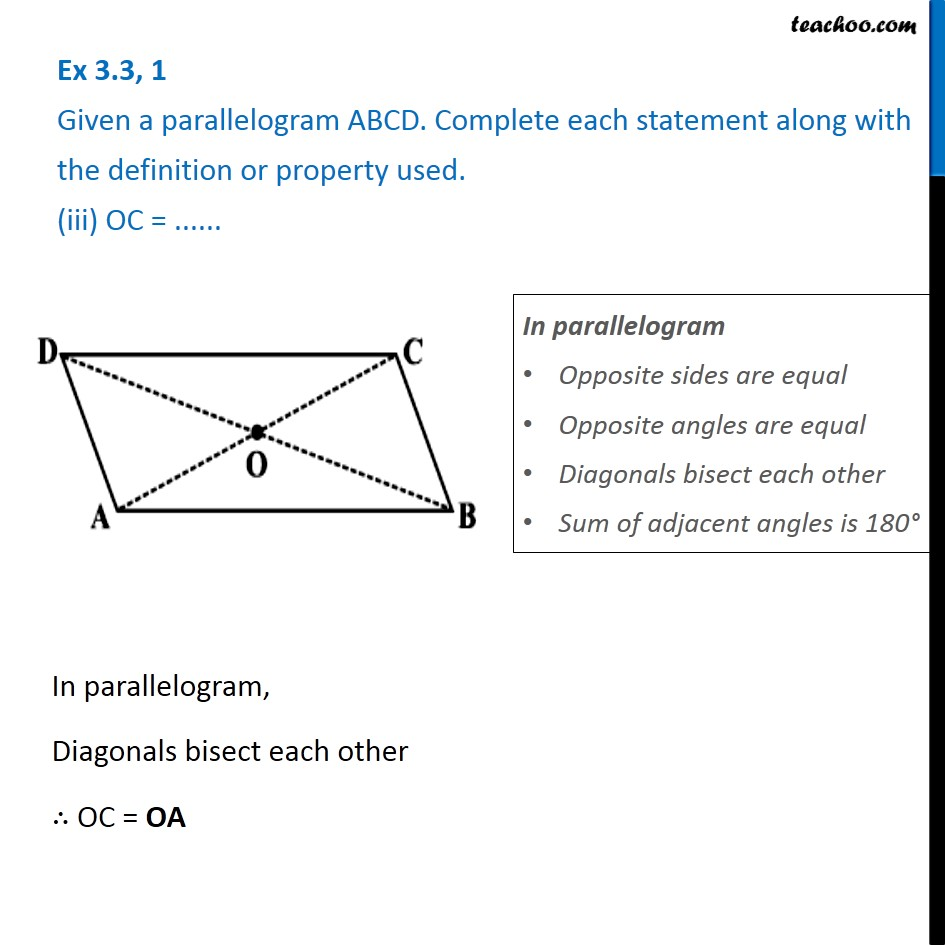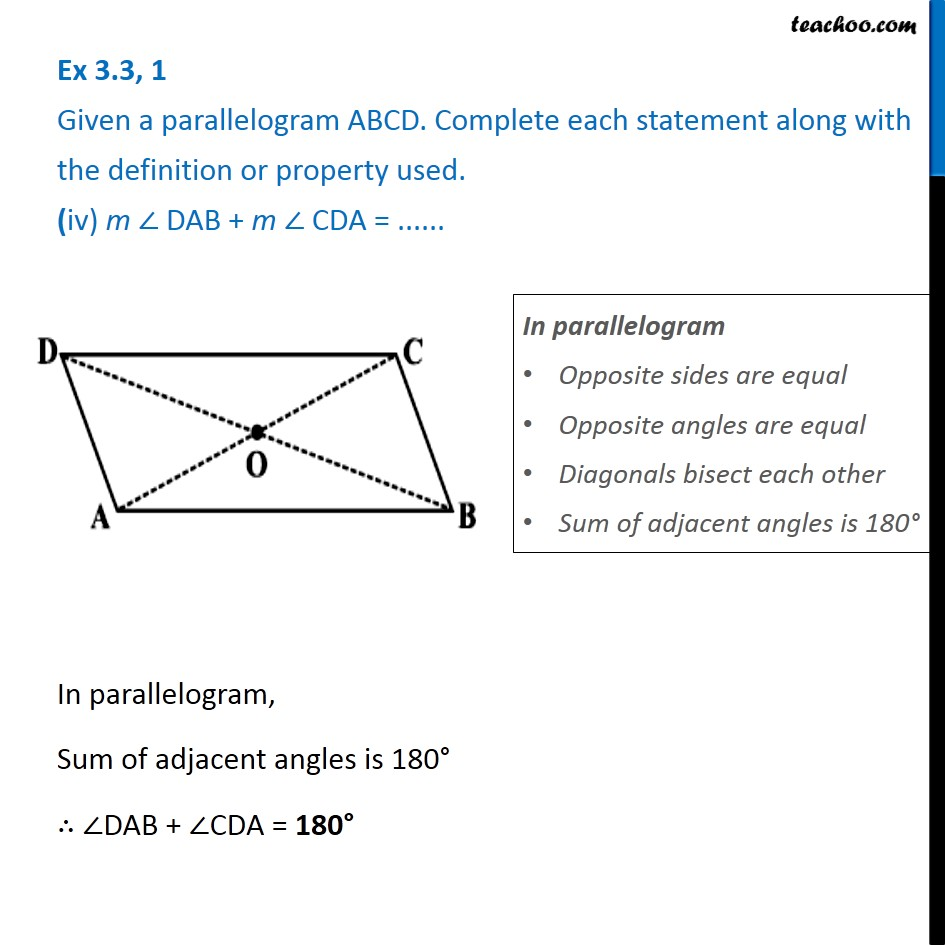Subscribe to our Youtube Channel - https://you.tube/teachoo

1. Chapter 3 Class 8 Understanding Quadrilaterals
2. Concept wise
3. Parallelogram

Transcript

Ex 3.3, 1 Given a parallelogram ABCD. Complete each statement along with the definition or property used. (i) AD = ......In parallelogram Opposite sides are equal Opposite angles are equal Diagonals bisect each other Sum of adjacent angles is 180° In parallelogram, Opposite sides are equal ∴ AD = BC Ex 3.3, 1 Given a parallelogram ABCD. Complete each statement along with the definition or property used. (ii) ∠ DCB = ...... In parallelogram Opposite sides are equal Opposite angles are equal Diagonals bisect each other Sum of adjacent angles is 180° In parallelogram, Opposite angles are equal ∴ ∠DCB = ∠BAD Ex 3.3, 1 Given a parallelogram ABCD. Complete each statement along with the definition or property used. (iii) OC = ......In parallelogram, Diagonals bisect each other ∴ OC = OA In parallelogram Opposite sides are equal Opposite angles are equal Diagonals bisect each other Sum of adjacent angles is 180° Ex 3.3, 1 Given a parallelogram ABCD. Complete each statement along with the definition or property used. (iv) m ∠ DAB + m ∠ CDA = ......In parallelogram Opposite sides are equal Opposite angles are equal Diagonals bisect each other Sum of adjacent angles is 180° In parallelogram, Sum of adjacent angles is 180° ∴ ∠DAB + ∠CDA = 180°

Parallelogram